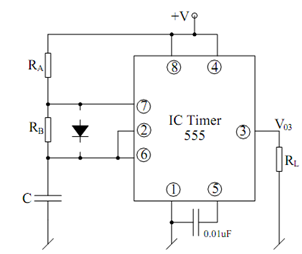## Basic Astable Multivibrator Assignment Help

Assignment Help: >> IC Timer - Basic Astable Multivibrator

Basic Astable Multivibrator:

An astable multivibrator is a relaxation oscillator, which does not have any permanent stable state. It alternates among two quasi-stable states and is, hence, appropriate for generating a square or rectangular waveform. Such circuits may be made from two BJTs, inverters, op-amps or IC Timer 555. We illustrates here how it may be done using IC 555 Timer.Figure: Basic Astable Multivibrator using 555 Timer

The operation of the circuit may be explained as follows. Suppose V03 to be HIGH, so that pin 7 behaves as open and C charges with a time constant (RA + RB) C towards + V. While Vc (t) becomes > 2/3 Vcc, V03 goes Low, pin 7 is short and at which instant, capacitor discharges through 7 with the time constant RB C till Vc (t) becomes <  (1/3)Vcc at which instant, Vo3 again goes high  .This sequence goes on repeating such that Vc (t) is confined between (1/3) Vcc and 2/3 Vcc and V03 alternates among High and Low as illustrated in the diagram. Since charging and the discharging times are not the similar, apparently, TH ≠ TL. Also, note down that (TH)1st cycle ≠ (TH) subsequent cycles and therefore, the circuit has 'first cycle timing error'.